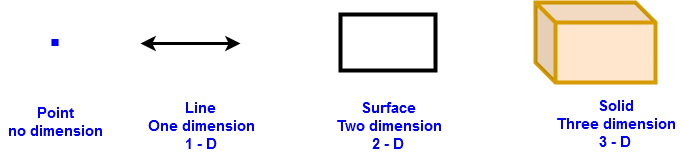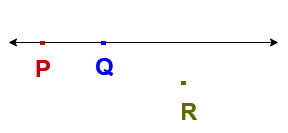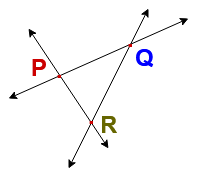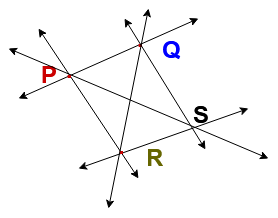# Point in Geometry Math | Collinear Points and non-collinear points Examples

In this article explained about defections of point in geometry math, Collinear Points, Non-collinear points with examples.

## Point in Math | Collinear Points | Non-collinear points | All Math Tricks

The terms Point, Line, Plane and space .. etc are fundamental concepts in study of geometry and they

### Definition of Point in Math:

A point is that which has no part. It has only one position. It does not have dimensions like length, breadth or height

Consider the step from points to solid

Point – Line – Surface – SolidsIn the above figures, the first figure is Point. It has no dimension. If it further add one dimension length then it will be line segment (Line). If further add one more dimension breadth then it will have two dimension which is rectangle(Surface) . Now add one more dimension height then it has three dimension which is cuboid (Solid).

### Collinear Points & non-collinear points:

Collinear points : Three or more points lying on the same line are called collinear points.

Non-collinear points : Three or more points are not lying on the same line are called non-collinear points.

Examples

Let us considered three points P, Q and R in a plane. If we draw a linel passing through two points P & Q , then there are two possibilities

a) Point R lies on the line l

b) Point R does not lie on the line lIf a point R lies on the line l then points P , Q &  R lie on the same line and are said to be collinear points.If a point R does not lie on the line l , then points P, Q and R do not lie on the same line and are said to be non- collinear points.

### Lines through non- collinear points

Examples of non-collinear points

Let consider P, Q and R are non- collinear points thenNumber of lines through P, Q and R non-collinear points = 3  (,,)

Let consider P, Q, R & S are non- collinear points thenNumber of lines through P, Q , R & S non-collinear points = 6 (,,,)

Number of lines through “n” non-collinear points =Related Topics

Two dimensional shapes formulas.

Quadrilateral Properties | Trapezium, parallelogram, Rhombus

Types of Triangles With examples | Properties of  Triangle

Properties of  circle in math |  Arc, Perimeter, Segment of circle

Thanks for reading. I Hope you liked this article “Point in Math | Collinear Points | Non-collinear points”.  Give feed back, comments and please don’t forget to share it.